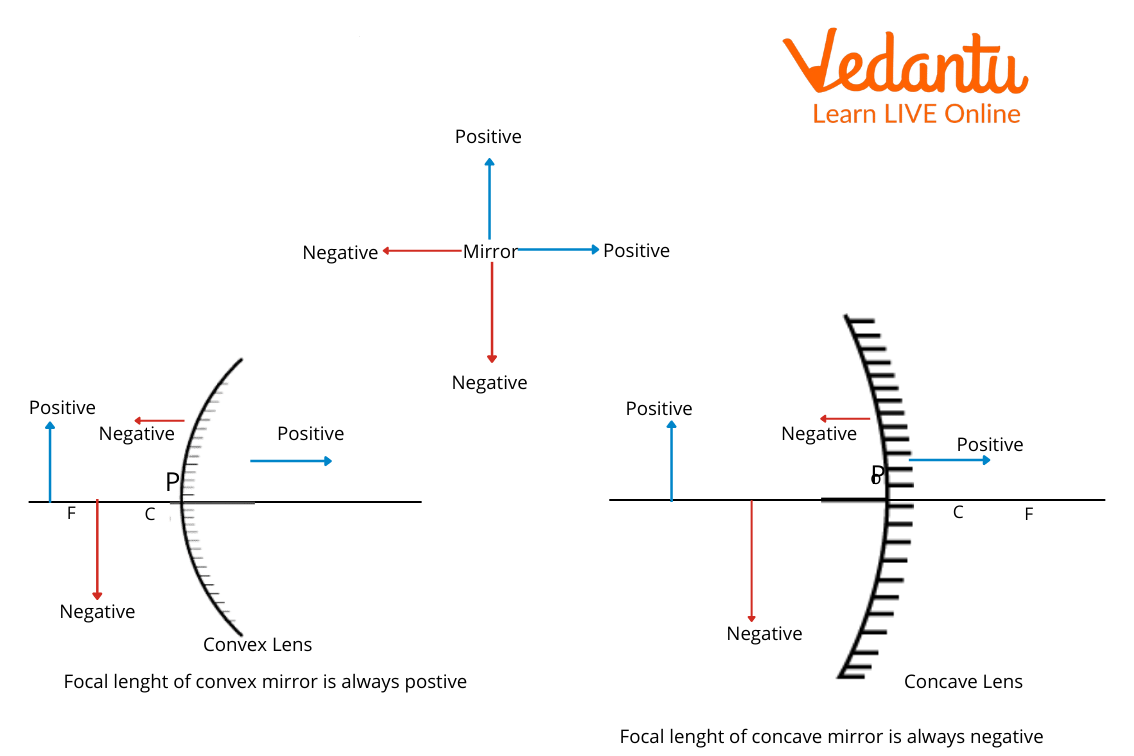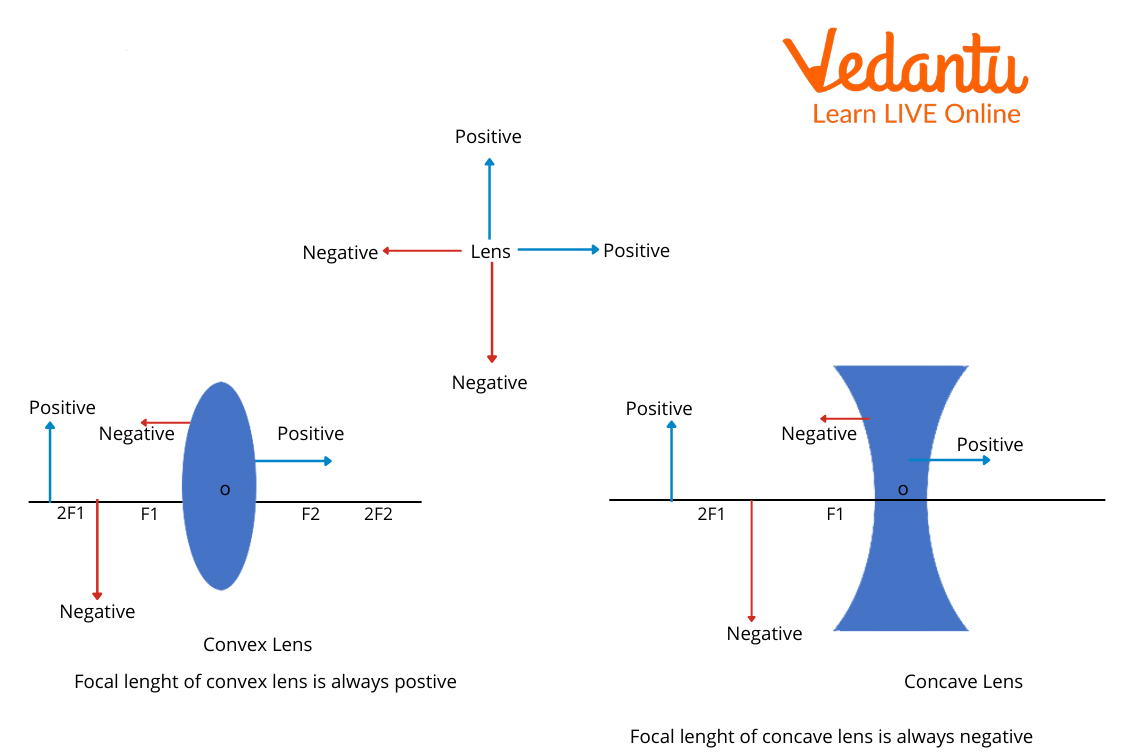Courses
Courses for Kids
Free study material
Free LIVE classes
More

# Sign Convention for MirrorsLIVE
Join Vedantu’s FREE Mastercalss

## Sign Convention in Spherical Mirrors: An Overview

Sign convention is nothing complicated but a simple way of making calculations simpler and easier by specifying certain rules while dealing with calculations for spherical mirror problems. These rules are a bit different when it comes to dealing with lenses, which we will be discussing later in this article as well. During sign convention application, we should always keep in mind what we are using them for since we already know that these will be different for lenses and spherical mirrors. In sign convention, we assign areas around our lens or mirror, a positive or negative sign and do our further calculations accordingly, keeping in mind the sign allocated to a particular region.

## What is a Sign Convention?

The sign convention is allocating positive or negative signs to regions around the mirror or lens, i.e., left or right and up or down of the principal axis of the mirror or lens, in order to make calculations easier and better to understand.

## Sign Convention for MirrorsSign Convention for Mirror

1. The distances which are measured along the direction of the incident ray are taken as positive.

2. The distances which are measured opposite the direction of the incident ray are taken as negative.

3. The region above the principal axis is considered positive.

4. The region below the principal axis is considered negative.

5. All measurements should be taken from the Pole of the mirror.

From the above points, we can conclude about Sign convention for spherical mirrors that:

1. The focal length of the concave mirror will always be negative since it is on the left of the mirror (in front of the mirror). Similarly, the focal length of the convex mirror will always be positive since it is on the right side of the mirror (behind the mirror).

2. Object distance will also be negative.

3. If a real image is formed, then it will form in front of the mirror, hence it will be negative.

4. If a virtual image is formed, then it will be formed behind the mirror, hence it will be taken as positive.

5. Since the object is always taken above the principal axis, we will take the object height as positive.

6. If the image formed is erect, then it will be above the principal axis, therefore its height should be taken as positive.

7. If the image formed is inverted, then it is formed below the principal axis, hence the image height should be taken as negative.

## Sign Convention for LensesSign Convention for Lens

1. The region on the right of the principal axis of the lens is positive.

2. The region on the left of the principal axis of the lens is negative.

3. The region above the principal axis is considered positive.

4. The region below the principal axis is considered negative.

5. All measurements should be taken from the Pole of the lens.

From the above convention, we can conclude that:

1. The focal length of a convex lens is always positive.

2. The focal length of a concave lens is always taken as negative.

3. The object taken is always in front of the lens, hence its distance will always be taken as negative.

4. If a real image is formed, then its distance will be taken as positive since it is being formed in the direction of the incident ray.

5. If a virtual image is formed, then its distance will be taken as negative since it is being formed opposite to the direction of the incident ray.

6. Coming to the object will be above the principal axis, hence take its height always as positive.

7. If the image is erect, then it will be above the principal axis, hence height will be taken as positive.

8. If the image formed is inverted, then it will be below the principal axis, hence it will be taken as negative.

## Some Important Questions

1. Can a real image be seen in the concave mirror?

Ans: When we place an object further away from the focal point of the mirror, a real image will be formed. But this image formed will be inverted.

1. What will be the sign convention for concave mirrors?

Ans: The image formed can be real or virtual in the case of the concave mirror. So, the image distance can be positive or negative. So, the focal length can also be positive or negative.

1. What will be the sign convention for convex lenses?

Ans: Convex mirrors always form virtual and erect images. So, for virtual images, the image distance is positive. And therefore, the focal length is also positive.

## Key Features

• We use sign convention to make our calculations and understanding easier.

• During calculations regarding mirror and lens, we should keep in mind the sign convention used to get correct results.

• The focus of the mirror and lens gets changed by changing the sign convention.

• Sign convention for focus is different for mirrors and lenses, hence it should be kept in mind whether dealing with a mirror or a lens.

• Finally, there are some important questions so as to clear our understanding of the topic.

Last updated date: 26th Sep 2023
Total views: 95.7k
Views today: 1.95k

## FAQs on Sign Convention for Mirrors

1. An object is placed 12 cm in front of a concave mirror which has a radius of curvature of 30 cm. Can you tell the characteristics of the image formed by the mirror?

We know that the object distance is 12 cm from the mirror and mirror curvature is 30 cm. To answer this question, we should know about image formation by a concave mirror. From this information, we can say that the object is placed between the focus and the pole; therefore, characteristics of the image formed by a concave mirror with the object placed between the focus and the pole will be:

• Virtual

• Enlarged

• Image will be formed behind the mirror

• Erect

2. What are the differences between concave and convex mirrors?

• Concave Mirror: Concave mirror is often known as a converging mirror because of its inward curve at the centre that reflects light inward, focusing at a single point. Depending upon the positions at which the object is placed from the mirror, a concave mirror can produce a variety of images.

• Convex Mirror: A convex mirror is known as a diverging mirror because of its outward bulge at the centre that allows light from different objects to fall directly on it. When parallel rays of light fall directly on the reflecting surface of the convex mirror, they diverge.

3. List some important uses of Mirrors and Lenses.

• Concave Lens: Used in cameras to improve the quality of photographs. It is also used to correct myopia which is also known as near-sightedness. It is also used as peepholes in doors for security purposes.

• Convex Lens: Important use of convex lenses is in the correction of hypermetropia or far-sightedness. It is also used in microscopes and magnifying glasses.

• Convex Mirror: It is used as reflectors in lamps used on streets. It also serves the purpose of the rearview mirror.

• Concave Mirror: It is used in Astronomical Telescope, as a reflector in headlights of vehicles and also as a shaving mirror.